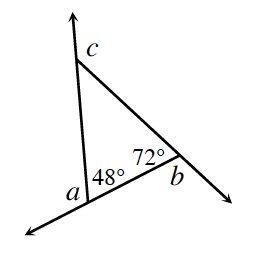### Home > GC > Chapter 7 > Lesson 7.2.5 > Problem7-84

7-84.$∠a$, $∠b$, and $∠c$ are exterior angles of the triangle at right. Find $m∠a$, $m∠b$, and $m∠c$. Then find $m∠a+m∠b+m∠c$.

How do the angles within a triangle relate to each other? How do the exterior angles relate to those within the triangle?